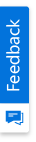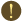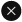We use cookies to give you the best experience on our website. If you continue to browse, then you agree to our privacy policy and cookie policy.Unfortunately, activation email could not send to your email. Please try again.# How to format the CalcQuick result?

Platform: WinForms |
Control: Calculate
Tags:

## Description

By default, the values from the particular key in CalcQuick are returned as an object value. This value can be converted as a string by using ToString() method. As separate support is not provided to format the result of the CalcQuick, the result is formatted as the following suggested way.

## Solution

To format the result of the CalcQuick calculations, the result can be parsed by using any of the formatting methods. For example,

1. int.Parse
2. double.Parse
3. decimal.Parse

C#

```private void calculateBtn_Click(object sender, EventArgs e)
{
//Reassigns the key values to the CalcQuick
calculator["A"] = this.value1Text.Text;
calculator["B"] = this.value2Text.Text;
calculator["C"] = this.formulaText.Text;
//Used to recalculate the all formulas in CalcQuick
calculator.SetDirty();
//Formats the result string after parsed as double
this.resultText.Text = double.Parse(calculator["C"].ToString()).ToString("#,##0.00");
}
```

VB

```Private Sub calculateBtn_Click(ByVal sender As Object, ByVal e As EventArgs) Handles calculateBtn.Click
'Reassigns the formula if changed
calculator("A") = Me.value1Text.Text
calculator("B") = Me.value2Text.Text
calculator("C") = Me.formulaText.Text
'Used to recalculate the all formulas in CalcQuick
calculator.SetDirty()
'Formats the result string after parsed as double
Me.resultText.Text = Double.Parse(calculator("C").ToString()).ToString("#,##0.00")
End Sub
```

Note:

The SetDirty() method is used for every calculations. I.e. whenever you are calculating the formulas with different values.

Refer to the following screenshot.2X faster development

The ultimate WinForms UI toolkit to boost your development speed.You are using an outdated version of Internet Explorer that may not display all features of this and other websites. Upgrade to Internet Explorer 8 or newer for a better experience.